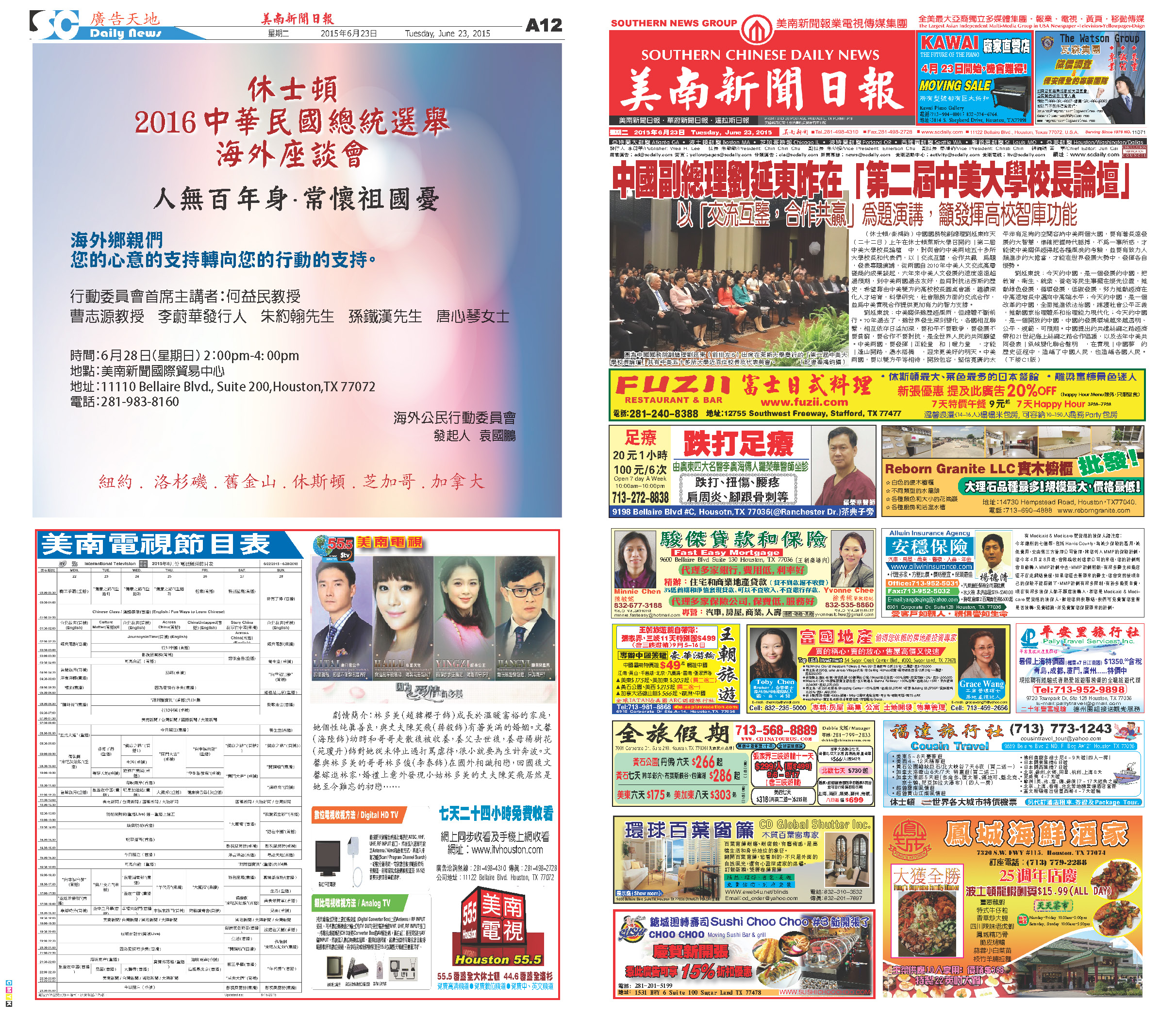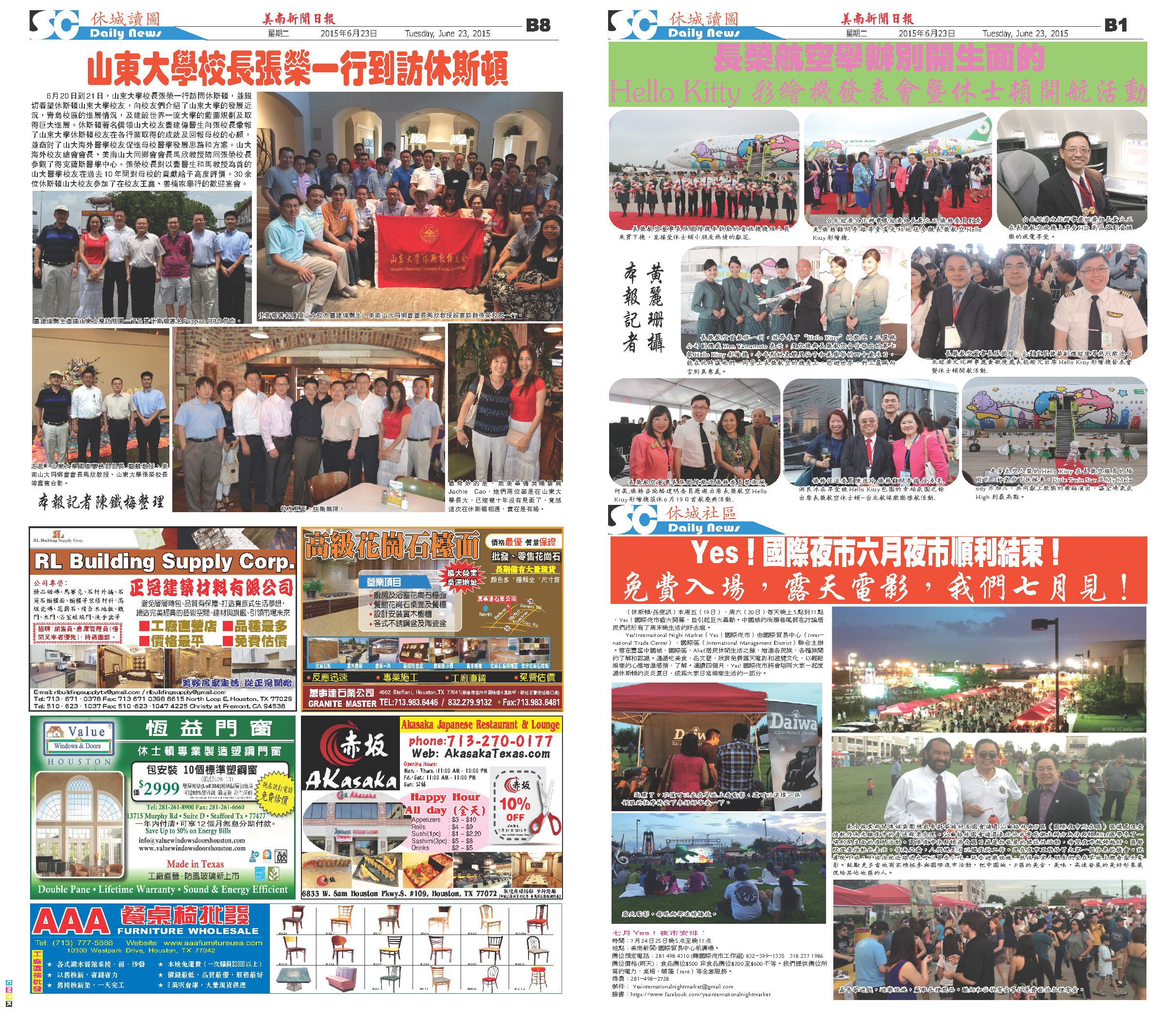150627 Epaper

 A Section B SectionC Section D Section150626 Epaper

 A Section B SectionC Section D Section150625 Epaper

 A Section B SectionC Section D Section150624 Epaper

 A Section B SectionC Section D Section150623 Epaper

 A Section B SectionC Section D Section150622 Epaper

 A Section B SectionC Section D Section150621 Epaper

 A Section B SectionC Section D Section150620 Epaper

 A Section B SectionC Section D Section150618 Epaper

 A Section B SectionC Section D Section150619 Epaper

 A Section B SectionC Section D Section150617 Epaper

 A Section B SectionC Section D Section